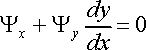# Integrating Factor

sleventh
Hello All,
Given the equation (2/y + y/x)dx + (3y/x + 2)dy
I am first asked to show the equation is not exact. To do this I showed the mixed partials were not equal i.e.:
(2/y + y/x)dy != (3y/x + 2)dx

I am then asked to find an integrating factor and show the potential function is given by
f = x^2 + y^3 + xy^2

I have consulted my ODE's text book but can not see how to apply the methods there to a multi-variable problem. I also haven't had much luck online.

Homework Helper
Gold Member
Hello All,
Given the equation (2/y + y/x)dx + (3y/x + 2)dy

That isn't an equation; it is just an expression. Perhaps you want to set it = 0.
I am first asked to show the equation is not exact. To do this I showed the mixed partials were not equal i.e.:
(2/y + y/x)dy != (3y/x + 2)dx

I am then asked to find an integrating factor and show the potential function is given by
f = x^2 + y^3 + xy^2

I have consulted my ODE's text book but can not see how to apply the methods there to a multi-variable problem. I also haven't had much luck online.

Try multiplying your equation by xy to clear the fractions. Also, since you are given a potential function, you could work backwards to see what to do.

sleventh
I see how multiplying by xy allows for an exact equation (and i think it's assumed to equal zero) since the mixed partials are equal. But to be honest, I do not see how you solve for the integrating factor other then guess by experience nor do I see how to actually solve for f(x,y) once you have your integrating factor. Thank you for any help.

Homework Helper
Gold Member
I see how multiplying by xy allows for an exact equation (and i think it's assumed to equal zero) since the mixed partials are equal. But to be honest, I do not see how you solve for the integrating factor other then guess by experience nor do I see how to actually solve for f(x,y) once you have your integrating factor. Thank you for any help.

Well, multiplying by xy is a no-brainer to get rid of the fractions. Sometimes if an equation is not exact, you can find an integrating factor that is a pure function of x or y. If you are interested, I printed an article about that that you can read as post #2 in the thread:

As to how to solve the equation once you have it exact, look at example 2 in

http://tutorial.math.lamar.edu/Classes/DE/Exact.aspx

for an easy to read example.

sleventh
That example was incredibly helpful. I was overcomplicating but now see it is in the factcan be written fromlooking at the single variable case i thought you had to use the e^int(p(x)) expression, but that is just a simple case used as example.

One last question i do not see how multiplying by a integrating factor allows for a general solution. Wouldn't you be changing your function by doing this?